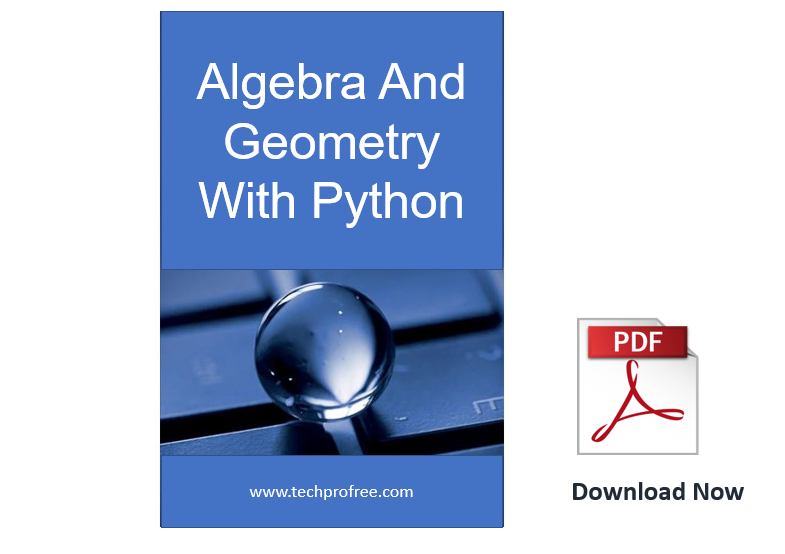Programming Books

# Learn Algebra and Geometry With Python PDF NotesDownload free Algebra And Geometry With Python in PDF. This Notes is very useful for advance undergraduate and graduate students in computer science. This notes teaches you Algebra and geometry. Source code of Python included in this notes. Main goal of these Notes is to bring together three topics

• Programming
• Math (Algebra & Geometery)
• Science

so within these notes you will be able to learn programmatically explore high school–level topics, like manipulating units of measurement; examining projectile motion

 Notes Algebra & Geometry With Python Type PDF Size 19 MB Language English For Beginners To Advance

You learn these topics from this notes:

1. Matrices And Matrix Algorithm

• Matrices and Operations With Them
• Concept of Algorithm, Correctness of Algorithm
• Estimation of Algorithm Efficiency
• Matrix Algorithm in the Graph Theory

2. Matrix Algebra

• Determinant of a Matrix: Determinant of a Second and Third Order
• Determinant of the n-th order: Minors
• Invers Matrix
• Integer Powers of a Matrix

3. Systems of Linear Equations

• Cramer’s Rules
• Invers Matrix Method
• Gaussian elimination

4. Complex Number and Matrices

• Athematic Operations with Complex Numbers
• Fundamental Theorem of Algebra
• Cardano Formula
• Fundamental of Quantum Computing

5. Vector Spaces

• Linear Dependence Vector of the Space
• Basis of the Space
• Euclidean Vector Space

6. Vector in a Three Dimensional Space

• Cartesian Coordinate System
• Scalar Product of Vector
• Vector Product of Vectors

7. Equation of a Straight Line on a Plane

• Slop-Intercept Form of the Equation of a Straight Line
• General Equation of Straight Line
• Equation of Straight Line Through Two Given Points

8. Equation of a Plane in Space

• General Equation of a Plane
• Intercept Form of the Equation of a Plane
• Normal Equation of a Plane

9. Equation of a Line in Space

• Angle Between Two Lines
• Angle Between Line and Plane
• Condition of Two Lines Belonging of a Plane

• Bilinear Forms
• Bringing the Quadratic Form to the Canonical  Form
• Jacobi Method

11. Curves of the Second Order

• Ellipse
• Hyperbola
• Parabola
• Degenerate curves

12. Elliptic Curves

• Operations of the Multiplication of the Elliptic Curves Points
• Addition of Two Different Points
• Duplication of a Point
• Elliptic Curves With Relational Points